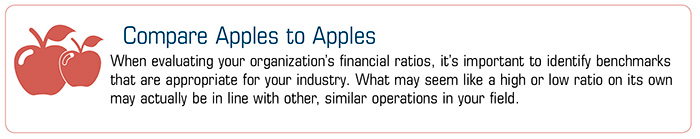#### Address

77 Water Street, 8th floor New York, NY 10005

#### Contact

Phone: 212 390 8918
Fax: 212 390 8913

#### E-mail

info@larreawealth.com

Address

Contact

E-mail

# Calculating Key Financial Ratios

Used to measure a business’s condition and performance, financial ratios can help you quantify your organization’s financial health and level of success. They are also used by those evaluating your business for potential investment or lending opportunities.

Your balance sheet and income statement will help you calculate the following widely used ratios.

Liquidity

Current ratio. This measures the amount of debt relative to assets (current assets divided by current liabilities). A current ratio of at least 1 (ideally, greater) indicates your business has enough assets to cover its short-term obligations.

Acid test or quick ratio. This ratio measures your organization’s ability to pay its current obligations with accessible assets. In other words, it helps you assess its “cash position.” The calculation is (cash and cash alternatives plus marketable securities plus accounts receivable) divided by current liabilities. The higher the ratio, the stronger the organization’s position.

Profitability

Gross profit margin. This ratio determines how much remains after accounting for the cost of goods sold (COGS) to pay for expenses, taxes, interest, etc. It is calculated by dividing gross profit by sales. (Gross profit equals sales minus COGS.)

Net profit margin. Net profit allows you to gauge how well your company is performing per dollar of revenue. It is calculated by dividing net income (income after expenses) by net revenue (revenue after adjusting for discounts and refunds).

Return on assets. Calculated by dividing net income by average total assets, this ratio shows the organization’s ability to generate income relative to overall assets. (To calculate average total assets, add the total assets at the beginning and end of the year and divide by two.)Activity

Accounts receivable turnover ratio. This ratio is used to evaluate the quality of receivables and to help determine how successful your organization is in collecting outstanding payments. It is determined by dividing net sales by average receivables outstanding over a given time period.

Inventory turnover ratio. This ratio can help determine whether your company is efficiently managing inventory. It is calculated by dividing COGS by average inventory (the average of the beginning and ending inventories over a period of time).

Leverage

Debt to asset ratio. This ratio measures the percentage of assets financed with debt rather than equity. The calculation is total debt divided by total assets.

Debt to equity ratio. This ratio compares an organization’s total debt to its total equity. The calculation is total liabilities divided by total equity. A high ratio may indicate a business has assumed a great deal of risk.